For reasons known only to ETS, number line problems are categorized with geometry. Such problems are basically algebra problems: they present you with some diagram or some facts about certain variables and then ask whether some equations or inequalities could be true, given certain relationships among the variables. Here is a straightforward example:

Example 1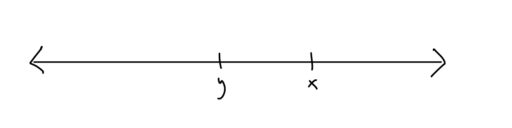x                         y
A.is greater thanB.is greater thanC. The two quantities are equal
D. It cannot be determined which, if any, is greater.

Now, a number line simply gives all the numbers from least to greatest. So the numbers to the right of the number line are greater than those to the left. And if there are markings on the number line, then you may (provided the question says nothing to the contrary) assume that the markings are evenly spaced. What does this mean? Well in the following diagram: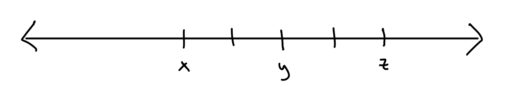you know that the distance betweenandis only half of the distance betweenand. Using algebra, we could express this as:Now, how do we solve number line problems? It will depend on what the question asks for. Some questions ask for which of the following could be true, whereas others ask about which of the following must be true.

If the questions asks which equations could be true, then we are looking for either a set of numbers that makes the equation true and is compatible with the diagram/given information, or we want to show that the equation is somehow inconsistent with our diagram/given information.

If the questions asks which equations must be true, then we are looking for either a set of numbers that is compatible with the diagram/given information but makes our equation false, or we want to show that the equation somehow follows from the diagram/given information.

Whether you should look for a specific example or some proof of the consistency/inconsistency of the given equation is a judgment call that you need to make in the moment. It's hard to give general principles about when to do which, but after doing some practice problems and just thinking about the equation at hand, you should develop some sense of how to decide if an equation is consistent or not. For example:

Example 2
Letand. Must the following be true?After doing some practice problems, you get a sense of which numbers to consider when you come to one of these problems. And by running through those numbers quickly, you can often show that some equation either can be satisfied (which answers whether the equation could be true) or can be violated (which answers whether the equation must be true).

The only real piece of advice I have here is two-fold: First, always try easy, concrete numbers. Don't just think about "one negative and one positive value." It's a lot more general to think that way, but also a lot harder to evaluate. Try, instead,orand. Nice, easy numbers to calculate. And if you want a small number, tryorSecond, it is easy to think about what happens if both are normal, natural numbers (e.g. 1 and 2). But also think about what happens if they are small, positive numbers (e.g.and) or only one is positive (e.g. 1 and -1) or both are negative (e.g. -1 and -2). If the equation works (or is always violated) in all of those cases, then probably it's always true (or always false).

When it comes to proving that some equation is either always true/false given the diagram/information in the question, look for easy inferences. And if you can't see a way to prove it quickly, consider just flagging it and coming back. If you've gone through a few diverse examples and they all come out the same way, probably the equation actually is always true (or always false) and the time spent confirming that might be better spent on other problems.

Practice Problems:

1. The markings on the below number line are evenly spaced: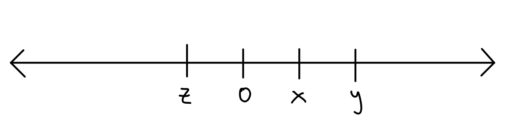Which of the following must be true (select all that apply):
A.B.C.2. Suppose we knowandWhich of the following must be true (select all that apply):
A.B.C.3. Suppose we knowWhich of the following must be true (select all that apply):
A.B.C.D.E.4. The markings in the below number line are equally spaced. Which of the following could be true: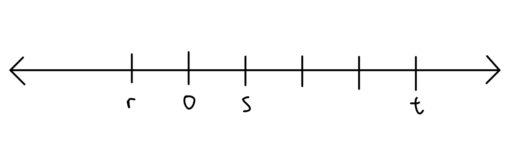A.B.C.5. The markings in the below number line are equally spaced. Which of the following must be true: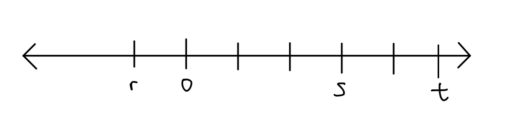A.B.C.